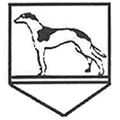# Maths

We have worked really hard to learn our number bonds for 10. How quickly can you say them? Can you use number bonds to 10 to find number bonds for 20?

We have been practising partitioning numbers into tens and ones.

We have used tensframes, bead strings, dienes and arrow cards to do this.

We have been solving problems using our knowledge of addition by combining the parts.

We have been using our knowledge of the part - part - whole model to solve missing addend equations.        2 + ? = 10

We have been learning addition by aggregation. This means representing a whole group split into parts. These parts are called the addend. We learned that the addends can go in any order and it does not change the sum of the parts together.

3    +     4     =   7                  4          +       3      =       7

We have been using the part - part - whole model to represent whole numbers. We did this first with cubes, then used pictures and then used numbers to represent this. We used the part - part - whole model in different orientations.

We have been showing our understanding of the place value of numbers by regrouping. Every time we got ten ones, we had to swap them for one group of ten.We have been representing numbers in different ways using different maths resources. See the gallery for more photos.